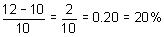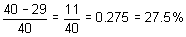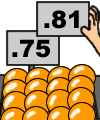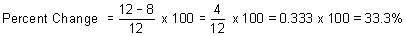# Calculating Percentage Increase And Decrease

## Learn About Calculating Percent Increase And Decrease With The Following Examples And Interactive Exercises.Example 1: Ann works in a supermarket for \$10.00 per hour. If her pay is increased to \$12.00, then what is her percent increase in pay?

Analysis: When finding the percent increase, we take the absolute value of the difference and divide it by the original value. The resulting decimal is then converted to a percent.

Solution:Answer: The percent increase in Ann's pay is 20%.

Let's look at an example of percent decrease.Example 2: The staff at a company went from 40 to 29 employees. What is the percent decrease in staff?

Analysis: When finding the percent decrease, we take the absolute value of the difference and divide it by the original value. The resulting decimal is then converted to a percent.

Solution:Answer: There was a 27.5% decrease in staff.

Percent increase and percent decrease are measures of percent change, which is the extent to which something gains or loses value. Percent changes are useful to help people understand changes in a value over time. Let's look at some more examples of percent increase and decrease.

In Example 1, we divided by 10, which was the lower number. In Example 2, we divided by 40, which was the higher number. Students often get confused by this. Remember that the procedure above asked us to divide by the original value. Another way to remember the procedure is to subtract the old value from the new value and then divide by the old value. Convert the resulting decimal to a percent. The formula is shown below.Example 3: At a supermarket, a certain item has increased from 75 cents per pound to 81 cents per pound. What is the percent increase in the cost of the item?

Solution:Answer: There was an 8% increase in the cost of the item.Example 4: Four feet are cut from a 12-foot board. What is the percent decrease in length?

Solution:Answer: There was a 33.3% decrease in length.

Summary: Percent increase and percent decrease are measures of percent change, which is the extent to which something gains or loses value. Percent change is useful to help people understand changes in a value over time. The formula for finding percent change is:### Exercises

Directions: Each problem below involves percent change. Enter your answer for each exercise without the percent symbol. Round your answer to the nearest tenth of a percent when necessary. For each exercise below, click once in the ANSWER BOX, type in your answer and then click ENTER. After you click ENTER, a message will appear in the RESULTS BOX to indicate whether your answer is correct or incorrect. To start over, click CLEAR.

 1.At Furnace Woods School, enrollment increased from 320 students in 2006  to 349 students in 2007. What is the percent increase in enrollment? ANSWER BOX:  %  RESULTS BOX:
 2.Stock in Company XYZ decreased from \$14 a share to \$9 a share.  What is the percent decrease in stock price? ANSWER BOX:  %  RESULTS BOX:
 3.The tuition at a college increased from 50,000 in 2006 to to 59,000 in 2007. What is the percent increase in tuition? ANSWER BOX:  %  RESULTS BOX:
 4.The price of oil decreased from \$54 per barrel to \$50 per barrel. What is the percent decrease in oil prices? ANSWER BOX:  %  RESULTS BOX:
 5.In a small town, the population increased from 25,000 people in 1990 to 32,000 people in 2000. What is the percent increase in population? ANSWER BOX:  %  RESULTS BOX: